Next: Partition Function Up: Applications of Statistical Thermodynamics Previous: System with Specified Mean

# Calculation of Mean Values

Consider a system in contact with a heat reservoir, or with a specified mean energy. The systems in the representative ensemble are distributed over their accessible states in accordance with the canonical distribution. Thus, the probability of occurrence of some statewith energy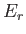is given by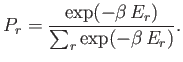(7.31)

The mean energy is written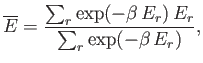(7.32)

where the sum is taken over all states of the system, irrespective of their energy. Note that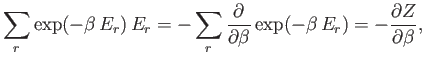(7.33)

where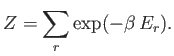(7.34)

It follows that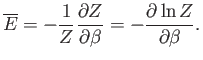(7.35)

The quantity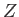, which is defined as the sum of the Boltzmann factor over all states, irrespective of their energy, is called the partition function. (Incidentally, the partition function is represented by the symbolbecause in German it is known as the zustandssumme,'' which means the sum over states.'') We have just demonstrated that it is fairly easy to work out the mean energy of a system using its partition function. In fact, as we shall discover, it is straightforward to calculate virtually any piece of statistical information from the partition function.

Let us evaluate the variance of the energy. We know that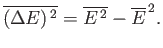(7.36)

(See Chapter 2.) Now, according to the canonical distribution,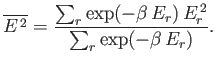(7.37)

However,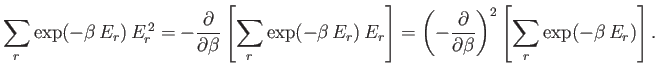(7.38)

Hence,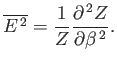(7.39)

We can also write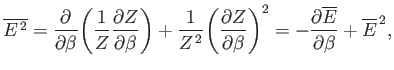(7.40)

where use has been made of Equation (7.35). It follows from Equation (7.36) that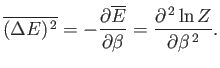(7.41)

Thus, the variance of the energy can be worked out from the partition function almost as easily as the mean energy. Because, by definition, a variance can never be negative, it follows that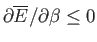, or, equivalently,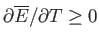. Hence, the mean energy of a system governed by the canonical distribution always increases with increasing temperature.

Suppose that the system is characterized by a single external parameter(such as its volume). The generalization to the case where there are several external parameters is straightforward. Consider a quasi-static change of the external parameter fromto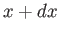. In this process, the energy of the system in statechanges by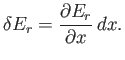(7.42)

The macroscopic work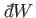done by the system due to this parameter change is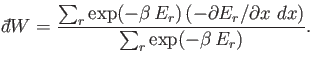(7.43)

In other words, the work done is minus the average change in internal energy of the system, where the average is calculated using the canonical distribution. We can write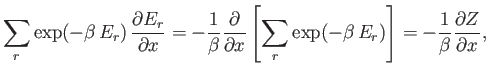(7.44)

which gives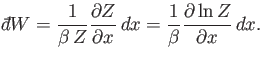(7.45)

We also have the following general expression for the work done by the system(7.46)

where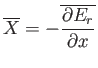(7.47)

is the mean generalized force conjugate to. (See Chapter 4.) It follows that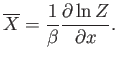(7.48)

Suppose that the external parameter is the volume, so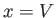. It follows that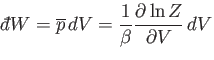(7.49)

and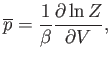(7.50)

whereis the pressure. Because the partition function is a function of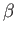and(the energiesdepend on), it follows that the previous equation relates the mean pressure,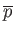, to(via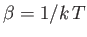) and. In other words, the previous expression is the equation of state. Hence, we can work out the pressure, and even the equation of state, using the partition function.Next: Partition Function Up: Applications of Statistical Thermodynamics Previous: System with Specified Mean
Richard Fitzpatrick 2016-01-25